# CSC 350 Learning Management Systems COMSATS Institute of

• Slides: 30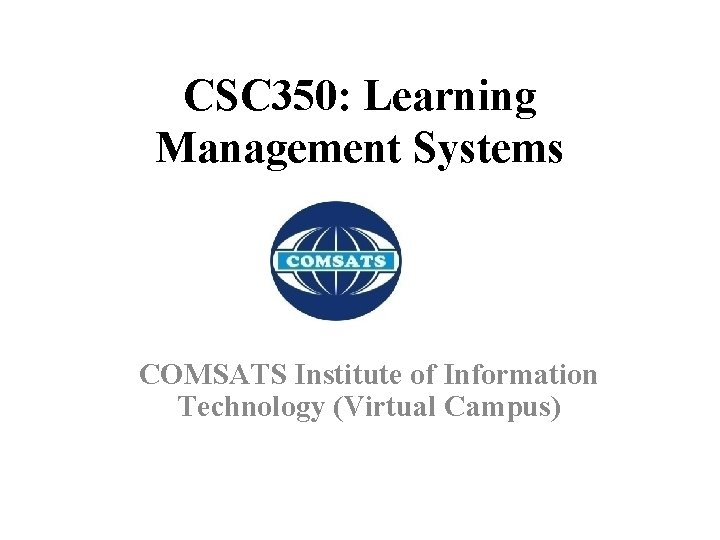CSC 350: Learning Management Systems COMSATS Institute of Information Technology (Virtual Campus)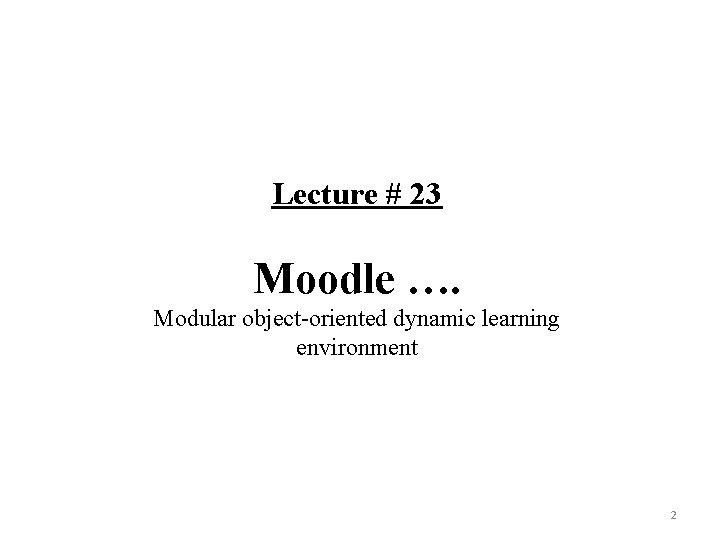Lecture # 23 Moodle …. Modular object-oriented dynamic learning environment 2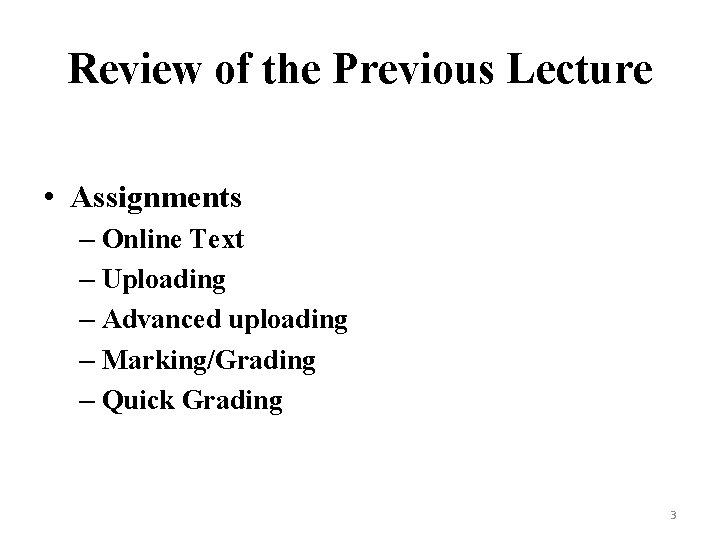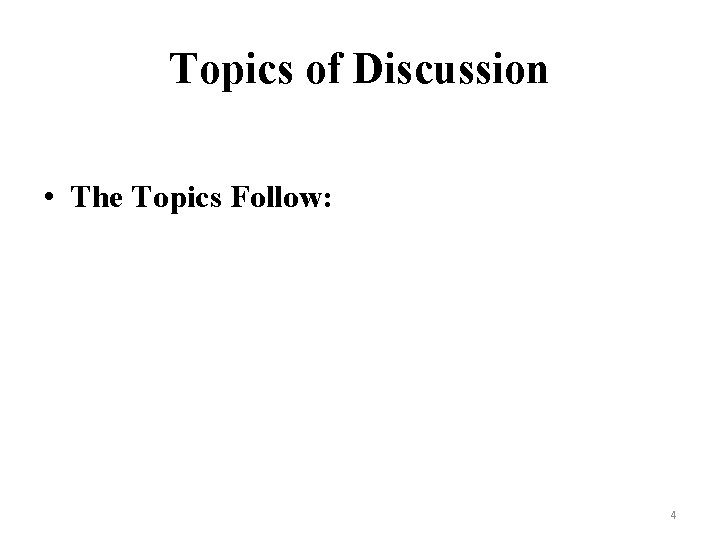Topics of Discussion • The Topics Follow: 4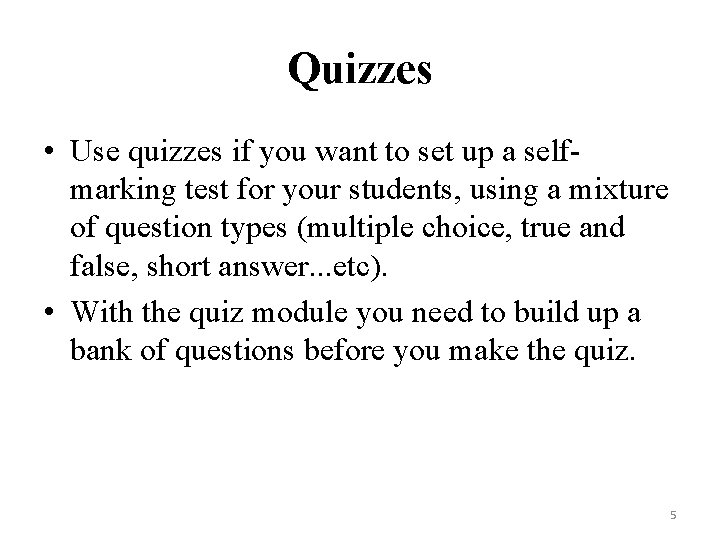Quizzes • Use quizzes if you want to set up a selfmarking test for your students, using a mixture of question types (multiple choice, true and false, short answer. . . etc). • With the quiz module you need to build up a bank of questions before you make the quiz. 5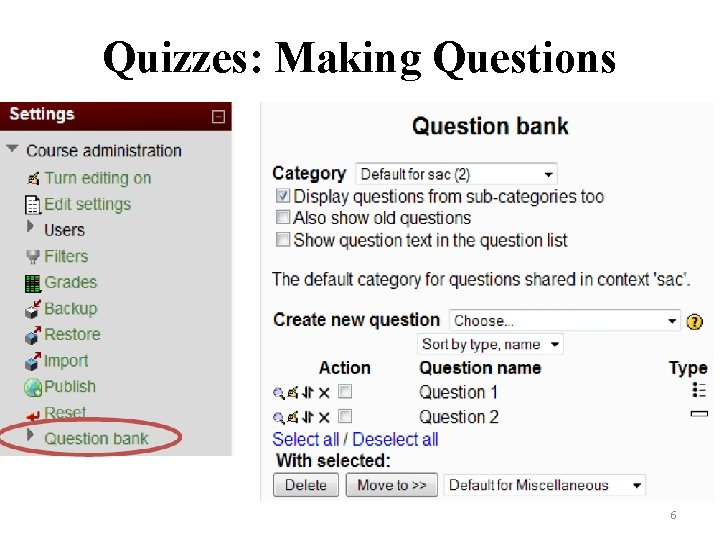Quizzes: Making Questions 6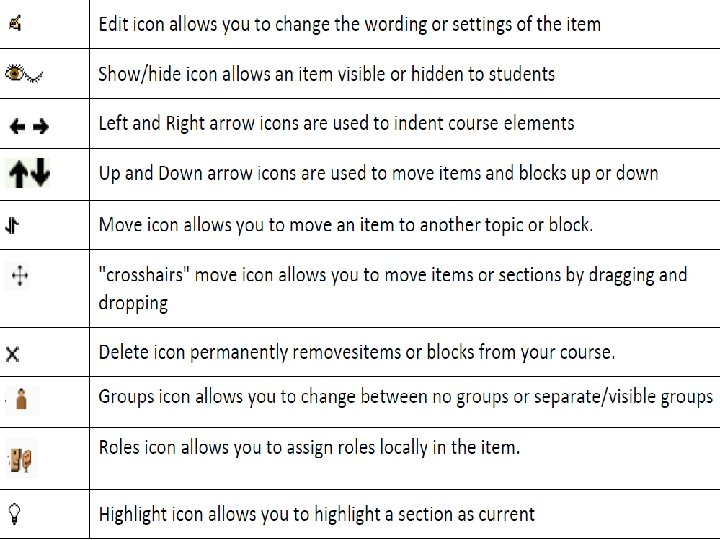7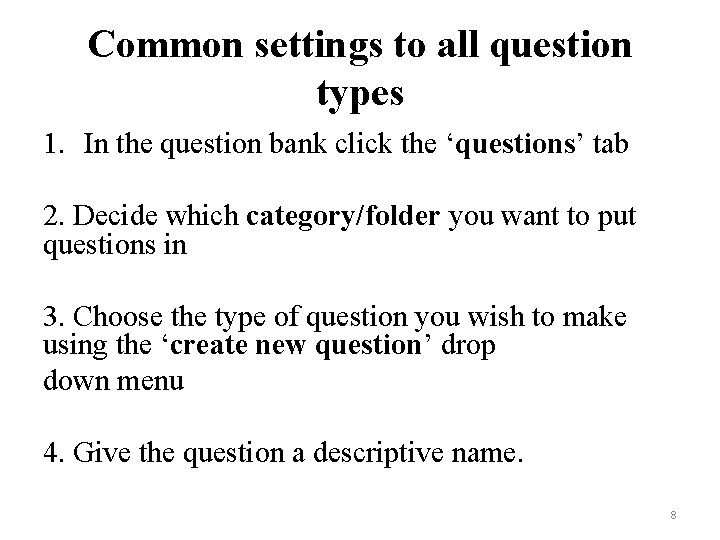Common settings to all question types 1. In the question bank click the ‘questions’ tab 2. Decide which category/folder you want to put questions in 3. Choose the type of question you wish to make using the ‘create new question’ drop down menu 4. Give the question a descriptive name. 8Common settings to … 5. Type the question into the ‘Question text’ box. What you type here will vary with the question type chosen (true/false, matching. . etc) 6. ‘Default question grade’ is the mark that will be awarded for a correct answer. 7. The ‘penalty’ factor is the mark that would be subtracted if the question was answered incorrectly and then reattempted. 9Common settings to … 8. When you set up a quiz you have an option to turn ‘adaptive mode’ on or off. Adaptive mode’ means students get a button to submit each answer individually (rather than answering all the questions and then submitting at the end). 9. General feedback allows you to give the same feedback to all students regardless of what answer they give. 10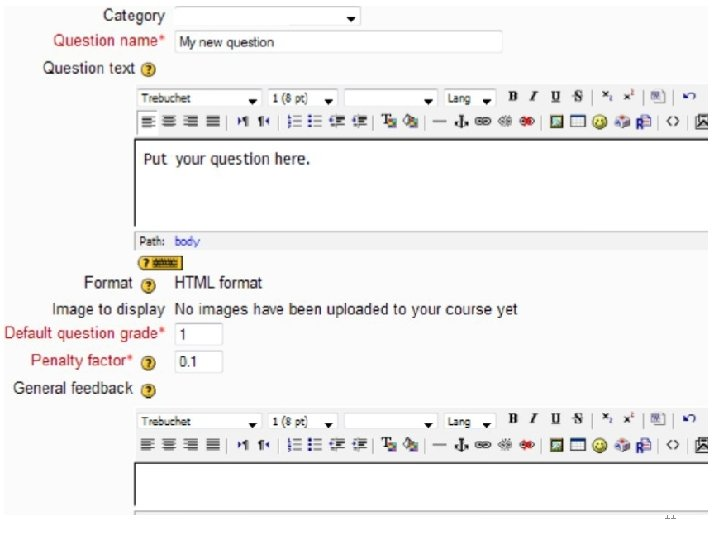11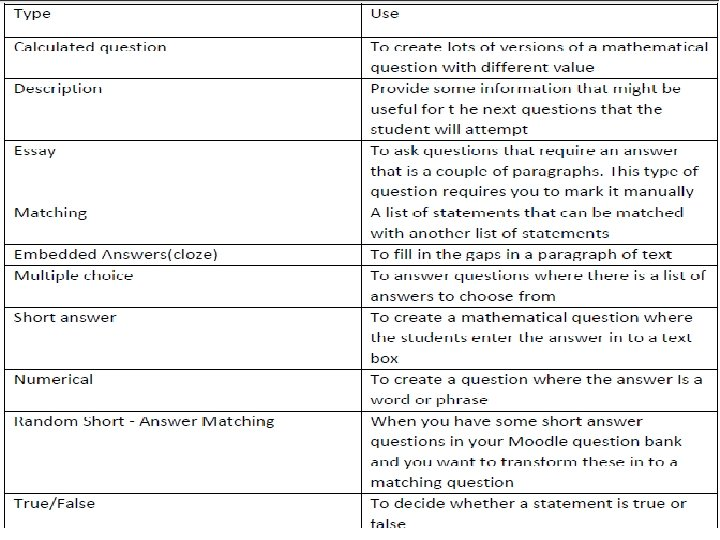12Description This can be used when you want to provide some info that might be useful for the questions. This is not a question – it’s like a label in the question bank. It would not work well if you chose to shuffle questions randomly. 1. Choose ‘description’ from the ‘Create new question’ drop down menu 2. Give the description a descriptive name 3. In the question text enter the information you want to give to students 4. Save Changes 13Description. . Essay Question This question type is used when you want to ask questions that require an answer that is no more than a couple of paragraphs. This type of question requires manual marking. Students’ grades will be 0 until you mark their answers. 1. Choose the ‘essay question’ from the ‘Create new question’ drop down menu 2. Give the question a descriptive name 14Description. . Essay Question 3. In the question text enter your question 4. Change the default grade to the number of marks the question is worth 5. If you want to give information regardless of the answer given, put that in the general feedback field. Note that this will appear once you have marked the question manually. Whatever you type in the feedback field will appear automatically when the question is submitted. 6. When you mark the question you also have a field for writing comments. 15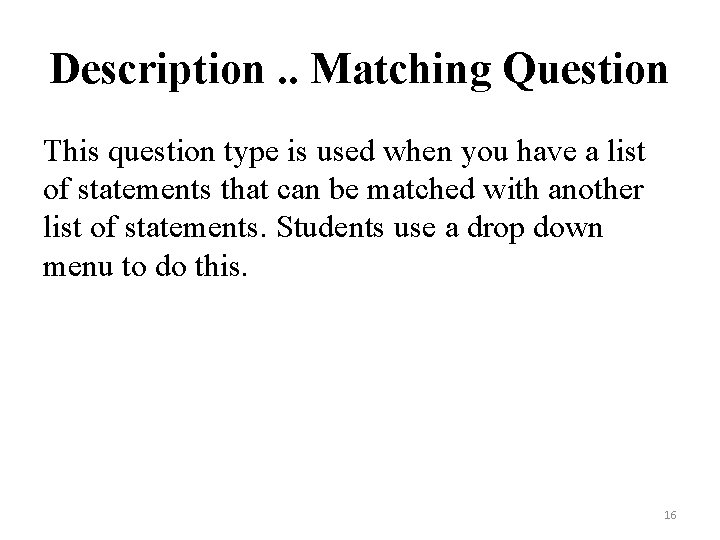Description. . Matching Question This question type is used when you have a list of statements that can be matched with another list of statements. Students use a drop down menu to do this. 16Matching Question 1. Choose ‘matching’ from the ‘Create new question’ drop down menu. 2. Give the question a descriptive name. 3. In the question text give instructions for the matching question. 17Matching Question 4. Ticking the ‘shuffle’ box means that the statements/questions on the right hand side (drop down menus) will appear in a random order each time the question is attempted. 5. Write statements/questions in the question field and the corresponding answer. The answers appear to students in a drop down menu. 6. Save changes 18Embedded Answers (Cloze) Question This question type is used when you want students to fill in the gaps in a paragraph of text. Students have to type into a box or choose from a drop down menu. 1. Choose ‘embedded answers (cloze)’ from the ‘Create new question’ drop down menu 2. Give the question a descriptive name 3. In the question text write your paragraph and then adapt it using Moodle code to produce different kinds of gaps. You can access reference information on this by clicking the question mark at the top of the page (alongside ‘Embedded answers’). 19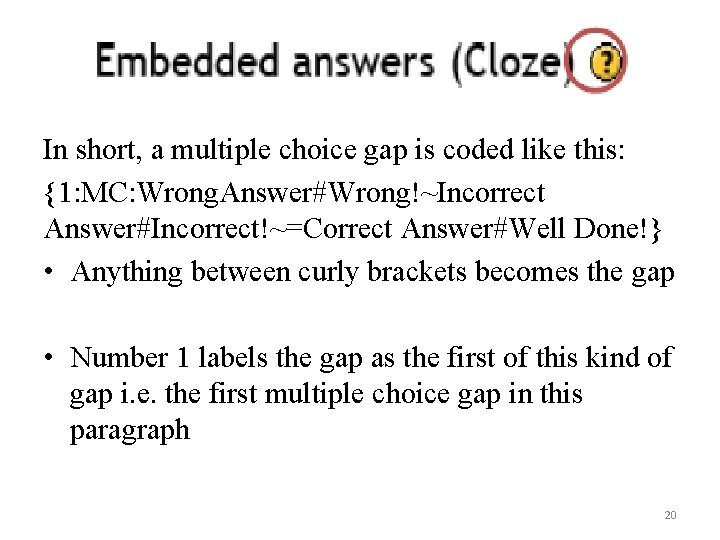In short, a multiple choice gap is coded like this: {1: MC: Wrong. Answer#Wrong!~Incorrect Answer#Incorrect!~=Correct Answer#Well Done!} • Anything between curly brackets becomes the gap • Number 1 labels the gap as the first of this kind of gap i. e. the first multiple choice gap in this paragraph 20• MC = multiple choice • The label, gap type and answers are separated by colons e. g. 1: MC: answers • Possible answers are separated by ~ • The correct answer is preceded by = • Feedback is separated from the answer by # 21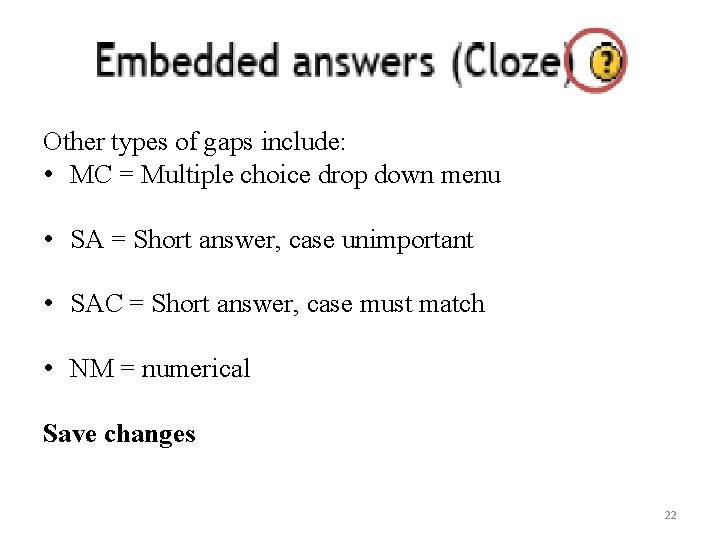Other types of gaps include: • MC = Multiple choice drop down menu • SA = Short answer, case unimportant • SAC = Short answer, case must match • NM = numerical Save changes 22Multiple Choice Questions 1. This question type is used when you want students to answer questions where there is a list of answers to choose from. There could be a single correct answer or several correct answers. 2. Choose ‘Multiple choice’ from the ‘Create new question’ drop down menu. 3. Give the question a descriptive name. 23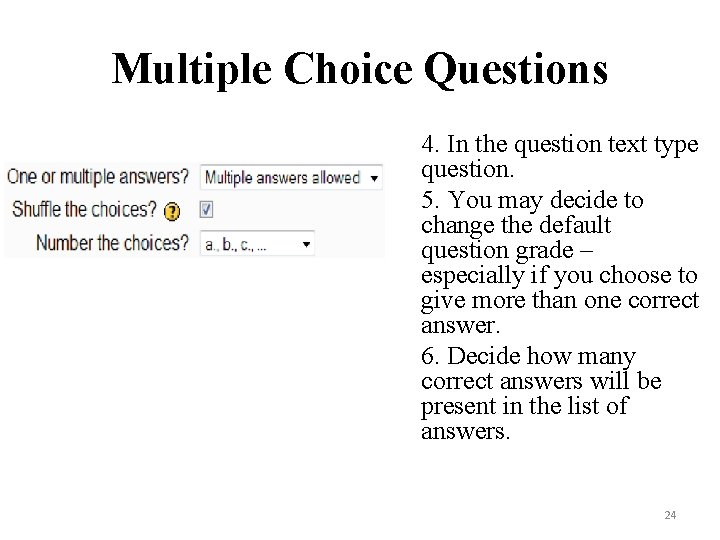Multiple Choice Questions 4. In the question text type question. 5. You may decide to change the default question grade – especially if you choose to give more than one correct answer. 6. Decide how many correct answers will be present in the list of answers. 24Multiple Choice Questions 7. Shuffling the choices is switched on by default so that the list of answers does not always appear in the same order. 8. You can change the way that choices are labeled by using the ‘Number the choices’ drop down menu 9. For each of the multiple choice answers (choices) you need to fill in the answer with the corresponding grade and feedback. It is possible to add more answers/choices by clicking ‘Blanks for 3 More Choices’ 25Multiple Choice Questions 10. The overall feedback section is useful because you can put feedback messages that the students see when they answer a given question correctly, partially correct or incorrectly. 11. Save Changes 26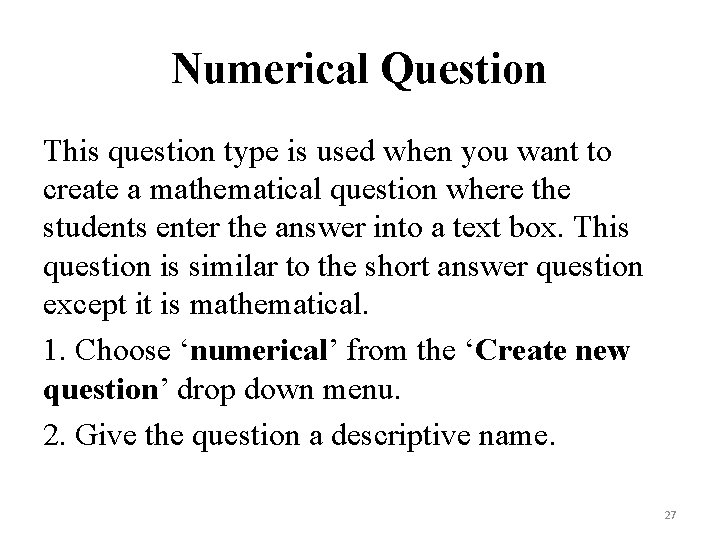Numerical Question This question type is used when you want to create a mathematical question where the students enter the answer into a text box. This question is similar to the short answer question except it is mathematical. 1. Choose ‘numerical’ from the ‘Create new question’ drop down menu. 2. Give the question a descriptive name. 27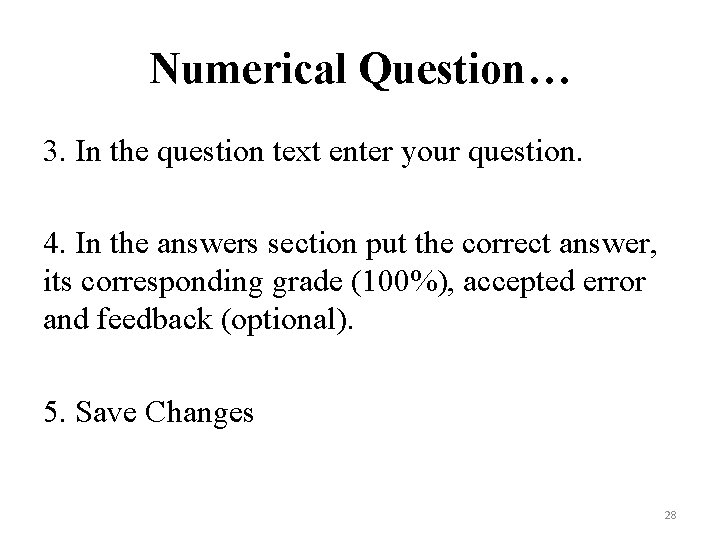Numerical Question… 3. In the question text enter your question. 4. In the answers section put the correct answer, its corresponding grade (100%), accepted error and feedback (optional). 5. Save Changes 28True/False Question This question type is used when you want students to decide whether a statement is true or false. 1. Choose ‘Short answer’ from the ‘Create new question’ drop down menu. 2. Give the question a descriptive name. 3. In the question text make a statement that is true or false. 4. Select whether the statement you wrote is true or false using the ‘correct answer’ drop down menu. 5. Give feedback corresponding to when students chose True or False. 6. Save Changes 29Thank You 30